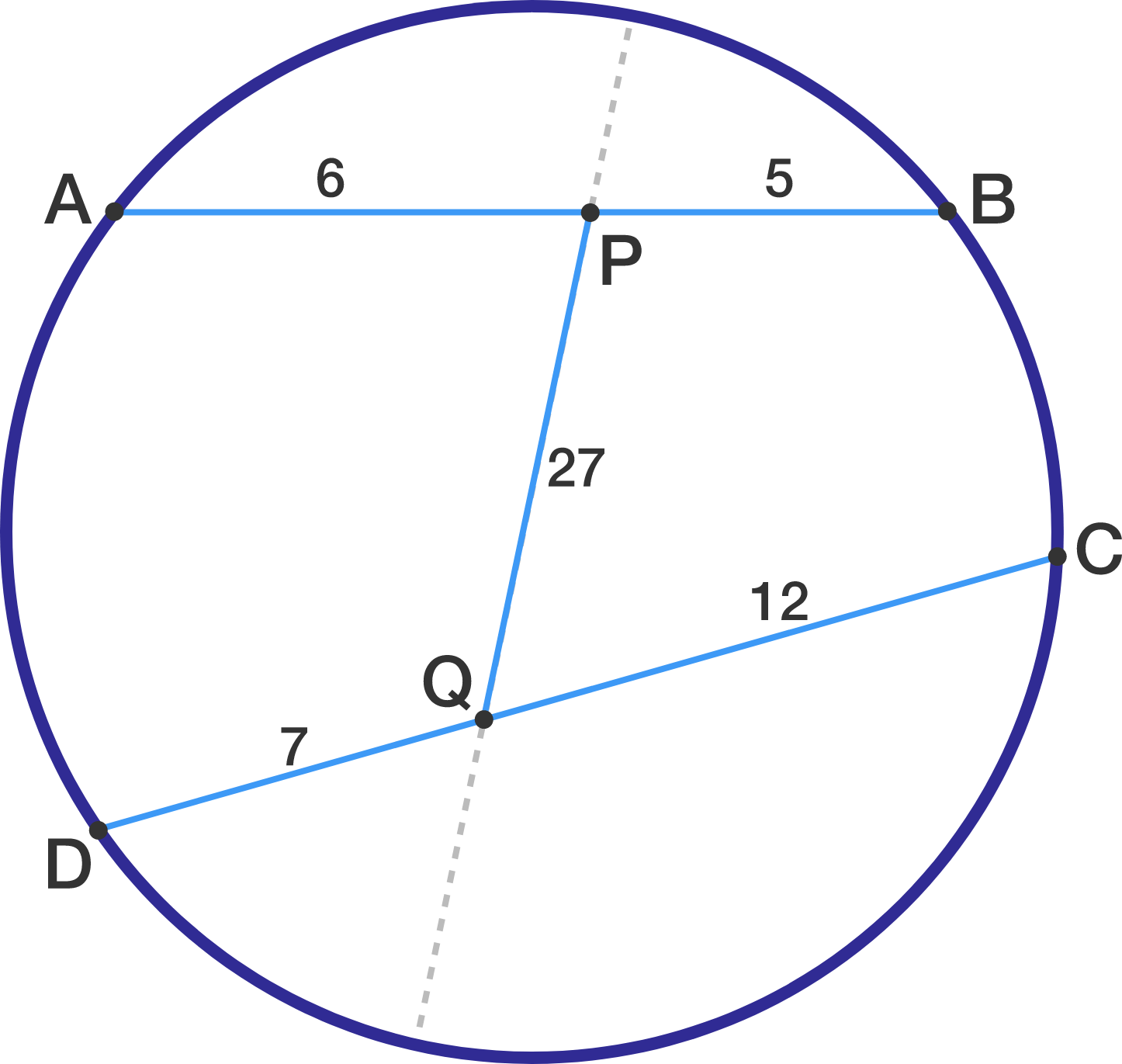$ABCD$ is a cyclic quadrilateral with $\displaystyle \overline{AB}=11$ and $\displaystyle \overline{CD}=19$. $P$ and $Q$ are points on $\overline{AB}$ and $\overline{CD}$, respectively, such that $\displaystyle \overline{AP}=6$, $\displaystyle \overline{DQ}=7$, and $\displaystyle \overline{PQ}=27.$ Determine the length of the line segment formed when $\displaystyle \overline{PQ}$ is extended from both sides until it reaches the circle.

Note: The image is not drawn to scale.

×